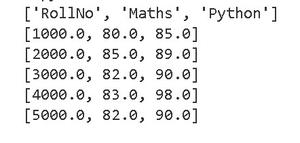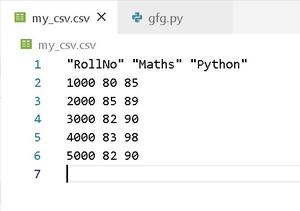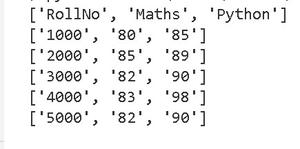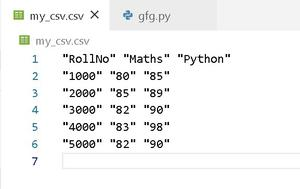Open In App

# How to read numbers in CSV files in Python?

Prerequisites: Reading and Writing data in CSV, Creating CSV files

CSV is a Comma-Separated Values file, which allows plain-text data to be saved in a tabular format. These files are stored in our system with a .csv extension. CSV files differ from other spreadsheet file types (like Microsoft Excel) because we can only have a single sheet in a file, and they cannot save cells, columns, or rows. Also, we cannot save formulas in this format.

To parse CSV files in Python, we make use of the csv library. The CSV library contains objects that are used to read, write and process data from and to CSV files. Let’s see how we can add numbers into our CSV files using csv library.

### Steps to read numbers in a CSV file:

1. Create a python file (example: gfg.py).
2. Import the csv library.
3. Create a nested-list ‘marks’ which stores the student roll numbers and their marks in maths and python in a tabular format.
4. Open a new csv file (or an existing csv file) in the ‘w’ mode of the writer object and other necessary parameters (here delimiter & quoting).
5. Write into it the list ‘marks’ with the help of writerows method.
6. In order to read the rows, make use of reader object and store each row(which is also a list) in a new list ‘output’.
7. Print the list output for verifying the code.

### Reading numbers in a CSV file without quotes:

In order to write in our CSV file ‘my_csv’,  we make use of the writerows() method of the writer object. But to read numbers as they are, we will make use of an optional parameter of the writer object, which is ‘quoting’. The ‘quoting’ parameter tells the writer which character is to be quoted.

If quoting is set to csv.QUOTE_NONNUMERIC, then .writerow() will quote all fields which contain text data and convert all numeric fields to the float data type.

Code:

## Python3

 `import` `csv` `# creating a nested list of roll numbers,``# subjects and marks scored by each roll number``marks ``=` `[``    ``[``"RollNo"``, ``"Maths"``, ``"Python"``],``    ``[``1000``, ``80``, ``85``],``    ``[``2000``, ``85``, ``89``],``    ``[``3000``, ``82``, ``90``],``    ``[``4000``, ``83``, ``98``],``    ``[``5000``, ``82``, ``90``]``]` `# using the open method with 'w' mode``# for creating a new csv file 'my_csv' with .csv extension``with ``open``(``'my_csv.csv'``, ``'w'``, newline ``=` `'') as ``file``:``    ``writer ``=` `csv.writer(``file``, quoting ``=` `csv.QUOTE_NONNUMERIC,``                        ``delimiter ``=` `' '``)``    ``writer.writerows(marks)` `# opening the 'my_csv' file to read its contents``with ``open``(``'my_csv.csv'``, newline ``=` `'') as ``file``:``  ` `    ``reader ``=` `csv.reader(``file``, quoting ``=` `csv.QUOTE_NONNUMERIC,``                        ``delimiter ``=` `' '``)``    ` `    ``# storing all the rows in an output list``    ``output ``=` `[]``    ``for` `row ``in` `reader:``        ``output.append(row[:])` `for` `rows ``in` `output:``    ``print``(rows)`

Output:output of gfg.py

And this is how it looks in the CSV file ‘my_csv.csv’ which gets created once we run the above code:my_csv.csv

### Reading numbers in a CSV file with quotes:

If quoting is set to csv.QUOTE_ALL then .writerow() will quote all fields and the numbers will now be stored in quotes. To read the numbers from each row, we make use of the reader object from CSV library and store all the rows within a list ‘output’, which we would also print afterward.

Code:

## Python3

 `import` `csv` `# creating a nested list of roll numbers,``# subjects and marks scored by each roll number``marks ``=` `[``    ``[``"RollNo"``, ``"Maths"``, ``"Python"``],``    ``[``1000``, ``80``, ``85``],``    ``[``2000``, ``85``, ``89``],``    ``[``3000``, ``82``, ``90``],``    ``[``4000``, ``83``, ``98``],``    ``[``5000``, ``82``, ``90``]``]` `# using the open method with 'w' mode``# for creating a new csv file 'my_csv' with .csv extension``with ``open``(``'my_csv.csv'``, ``'w'``, newline ``=` `'') as ``file``:``    ``writer ``=` `csv.writer(``file``, quoting ``=` `csv.QUOTE_ALL,``                        ``delimiter ``=` `' '``)``    ``writer.writerows(marks)` `# opening the 'my_csv' file to read its contents``with ``open``(``'my_csv.csv'``, newline ``=` `'') as ``file``:``    ``reader ``=` `csv.reader(``file``,``                        ``quoting ``=` `csv.QUOTE_ALL,``                        ``delimiter ``=` `' '``)``    ` `    ``# storing all the rows in an output list``    ``output ``=` `[]``    ``for` `row ``in` `reader:``        ``output.append(row[:])` `for` `rows ``in` `output:``    ``print``(rows)`

Output:output of gfg.py

And this how the above input gets stored within ‘my_csv.csv’ file:my_csv.csv